2018-10-30 22:19:36 Eastmount 阅读数 5593
• ###### 学习OpenCV3.2+QT5+ffmpeg实战开发视频编辑器视频教程

OpenCV3.2+QT5+ffmpeg实战开发视频编辑器视频培训课程概况：教程中会讲解到基于opencv视频和摄像机录制、播放和播放进度控制，多视频图像合并、多视频图像融合、剪切、视频亮度、对比度、尺寸（近邻插值（手动实现），双线性插值，图像金字塔）、颜色格式（灰度图，二值化（阈值）），旋转镜像，视频裁剪（ROI），视频水印（ROI+weight），导出处理后的视频（包含音频，使用ffmpeg工具对音频进行抽取、剪切和终于opencv处理的视频合并）。

19946 人正在学习 去看看 夏曹俊

PS：请求帮忙点个Star，哈哈，第一次使用Github，以后会分享更多代码，一起加油。

1.阈值化
2.二进制阈值化
3.反二进制阈值化
4.截断阈值化
5.反阈值化为0
6.阈值化为0

PS：文章参考自己以前系列图像处理文章及OpenCV库函数，同时部分参考网易云视频，推荐大家去学习。同时，本篇文章涉及到《计算机图形学》基础知识，请大家下来补充。

PSS：2019年1~2月作者参加了CSDN2018年博客评选，希望您能投出宝贵的一票。我是59号，Eastmount，杨秀璋。投票地址：https://bss.csdn.net/m/topic/blog_star2018/index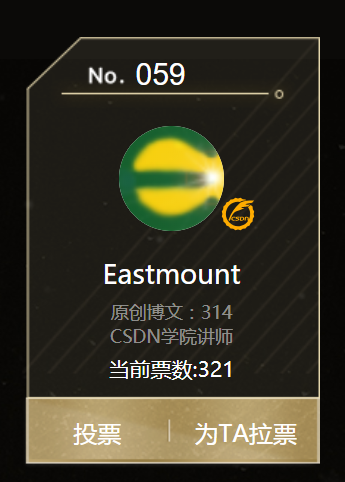# 一. 阈值化

（注：该部分参考作者的论文《基于苗族服饰的图像锐化和边缘提取技术研究》）

$\begin{cases} Y=0，gray=T\\ \end{cases}$

Python OpenCV中提供了阈值函数threshold()实现二值化处理，其公式及参数如下图所示：
retval, dst = cv2.threshold(src, thresh, maxval, type)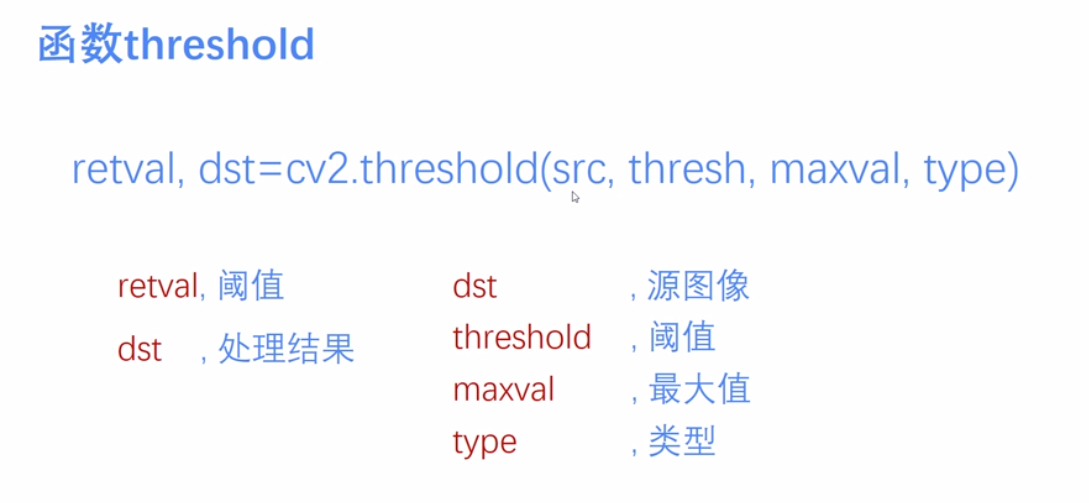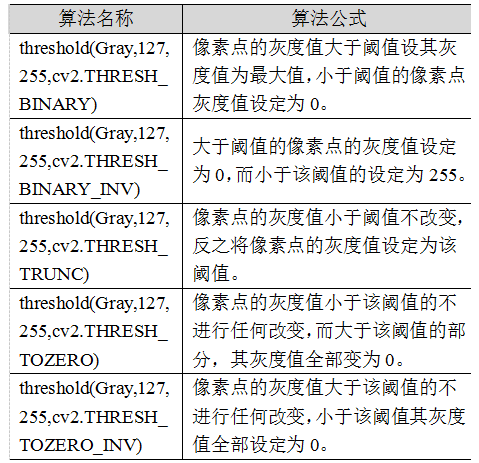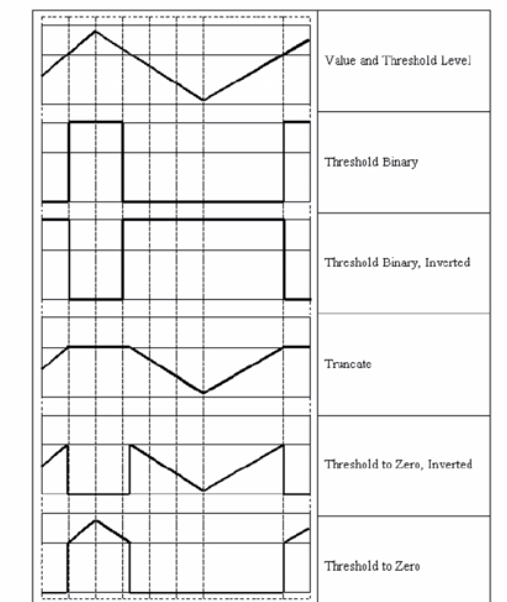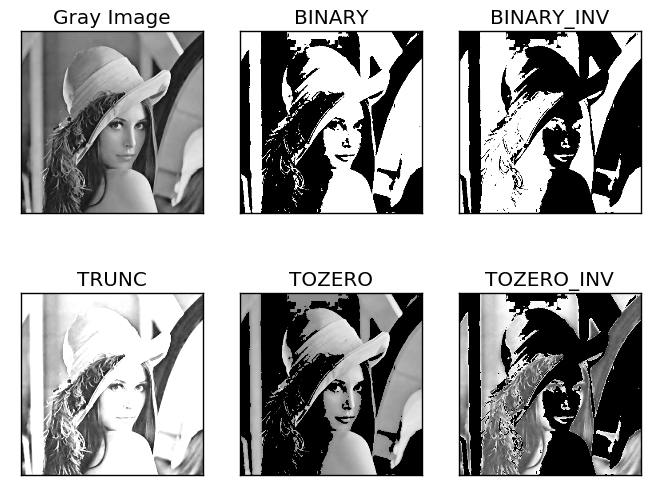# 二. 二进制阈值化

$dst(x,y) = \begin{cases} maxVal， if src(x,y)>thresh\\ 0，otherwise\\ \end{cases}$
(1) 大于等于127的像素点的灰度值设定为最大值（如8位灰度值最大为255）
(2) 灰度值小于127的像素点的灰度值设定为0

#encoding:utf-8
import cv2
import numpy as np

#读取图片

#灰度图像处理
GrayImage = cv2.cvtColor(src,cv2.COLOR_BGR2GRAY)

#二进制阈值化处理
r, b = cv2.threshold(GrayImage, 127, 255, cv2.THRESH_BINARY)
print r

#显示图像
cv2.imshow("src", src)
cv2.imshow("result", b)

#等待显示
cv2.waitKey(0)
cv2.destroyAllWindows()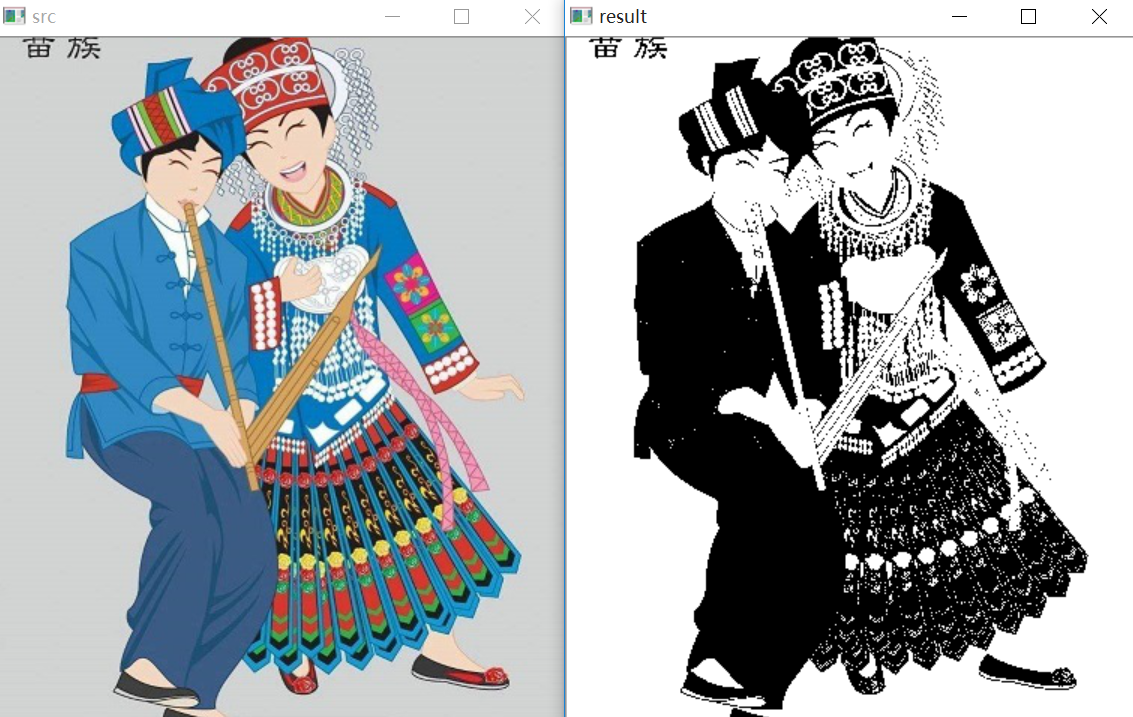# 三. 反二进制阈值化

$dst(x,y) = \begin{cases} 0， if src(x,y)>thresh\\ maxVal，otherwise\\ \end{cases}$
(1) 大于127的像素点的灰度值设定为0（以8位灰度图为例）
(2) 小于该阈值的灰度值设定为255

#encoding:utf-8
import cv2
import numpy as np

#读取图片

#灰度图像处理
GrayImage = cv2.cvtColor(src,cv2.COLOR_BGR2GRAY)

#反二进制阈值化处理
r, b = cv2.threshold(GrayImage, 127, 255, cv2.THRESH_BINARY_INV)
print r

#显示图像
cv2.imshow("src", src)
cv2.imshow("result", b)

#等待显示
cv2.waitKey(0)
cv2.destroyAllWindows()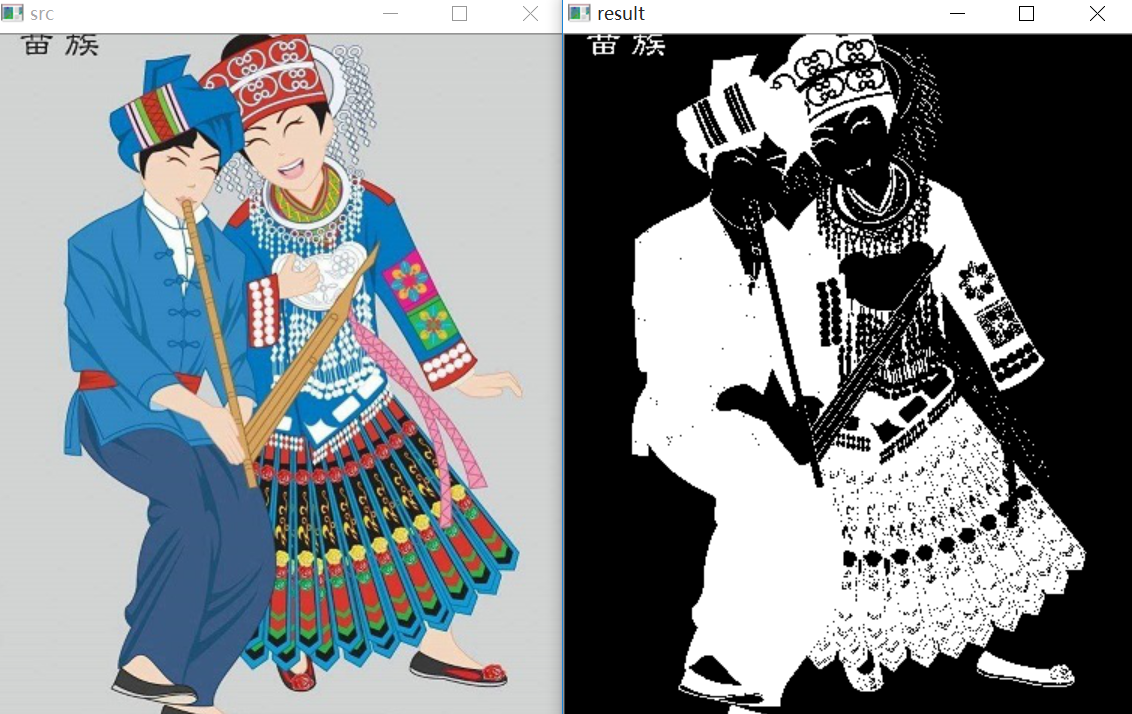# 四. 截断阈值化

$dst(x,y) = \begin{cases} threshold， if src(x,y)>thresh\\ src(x,y)，otherwise\\ \end{cases}$
(1) 大于等于127的像素点的灰度值设定为该阈值127
(2) 小于该阈值的灰度值不改变

#encoding:utf-8
import cv2
import numpy as np

#读取图片

#灰度图像处理
GrayImage = cv2.cvtColor(src,cv2.COLOR_BGR2GRAY)

#截断阈值化处理
r, b = cv2.threshold(GrayImage, 127, 255, cv2.THRESH_TRUNC)
print r

#显示图像
cv2.imshow("src", src)
cv2.imshow("result", b)

#等待显示
cv2.waitKey(0)
cv2.destroyAllWindows()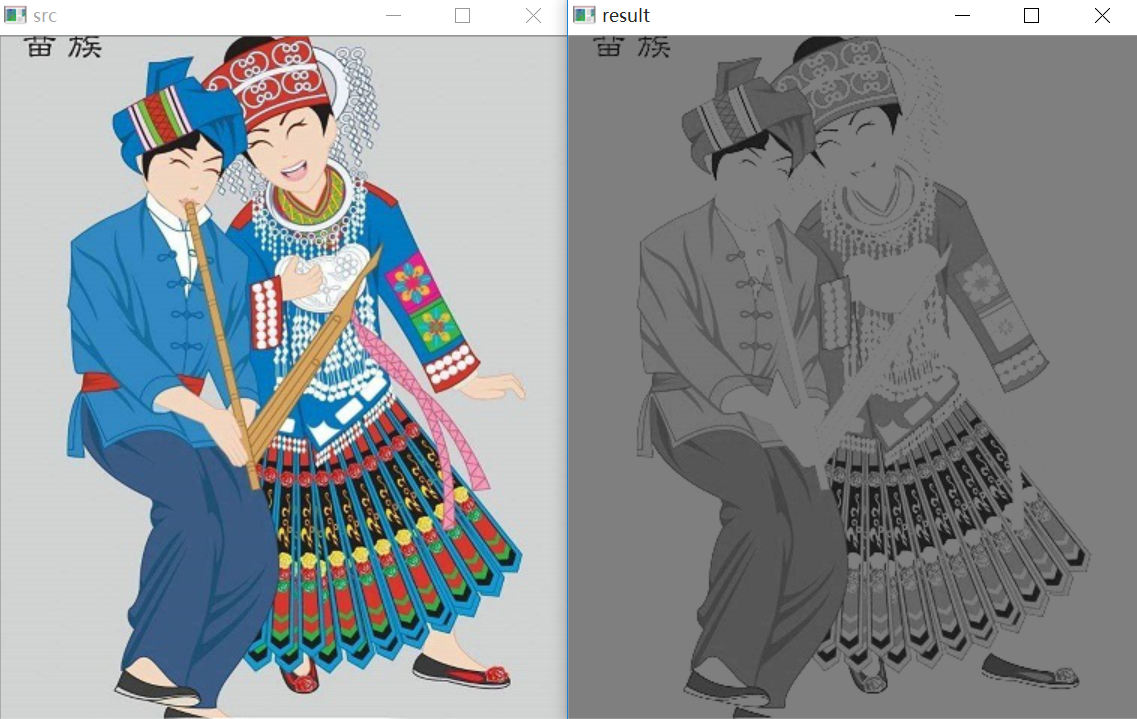# 五. 反阈值化为0

$dst(x,y) = \begin{cases} 0， if src(x,y)>thresh\\ src(x,y)，otherwise\\ \end{cases}$
(1) 大于等于阈值127的像素点变为0
(2) 小于该阈值的像素点值保持不变

#encoding:utf-8
import cv2
import numpy as np

#读取图片

#灰度图像处理
GrayImage = cv2.cvtColor(src,cv2.COLOR_BGR2GRAY)

#反阈值化为0处理
r, b = cv2.threshold(GrayImage, 127, 255, cv2.THRESH_TOZERO_INV)
print r

#显示图像
cv2.imshow("src", src)
cv2.imshow("result", b)

#等待显示
cv2.waitKey(0)
cv2.destroyAllWindows()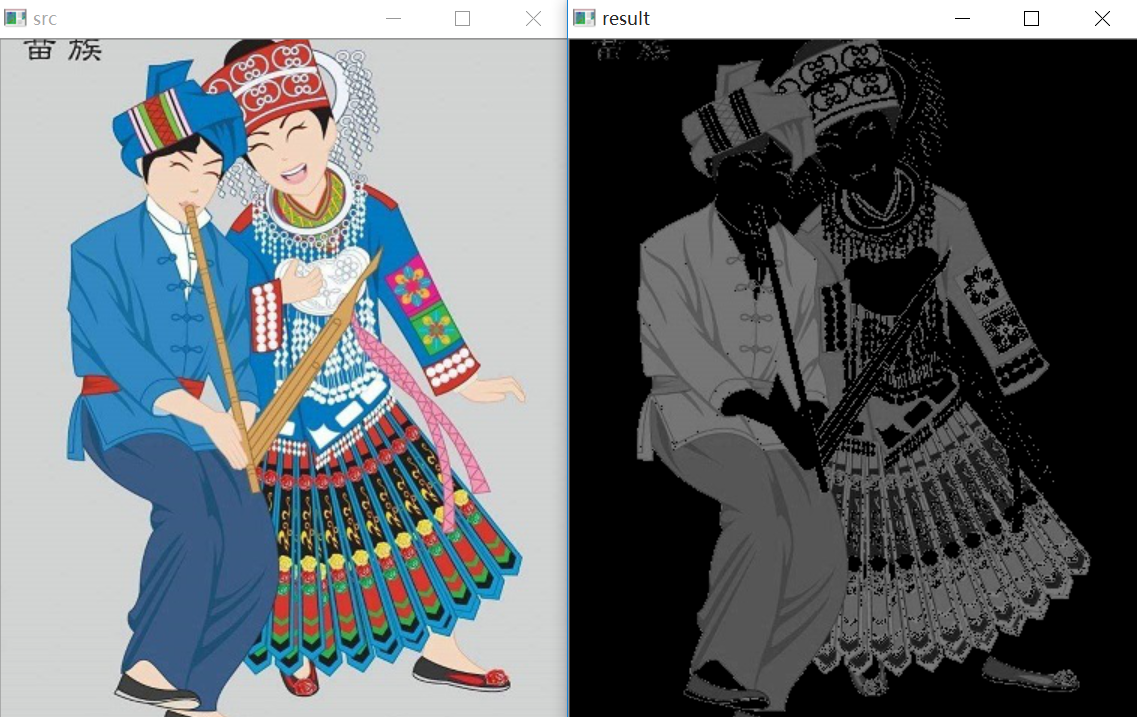# 六. 阈值化为0

$dst(x,y) = \begin{cases} src(x,y)， if src(x,y)>thresh\\ 0，otherwise\\ \end{cases}$
(1) 大于等于阈值127的像素点，值保持不变
(2) 小于该阈值的像素点值设置为0

#encoding:utf-8
import cv2
import numpy as np

#读取图片

#灰度图像处理
GrayImage = cv2.cvtColor(src,cv2.COLOR_BGR2GRAY)

#阈值化为0处理
r, b = cv2.threshold(GrayImage, 127, 255, cv2.THRESH_TOZERO)
print r

#显示图像
cv2.imshow("src", src)
cv2.imshow("result", b)

#等待显示
cv2.waitKey(0)
cv2.destroyAllWindows()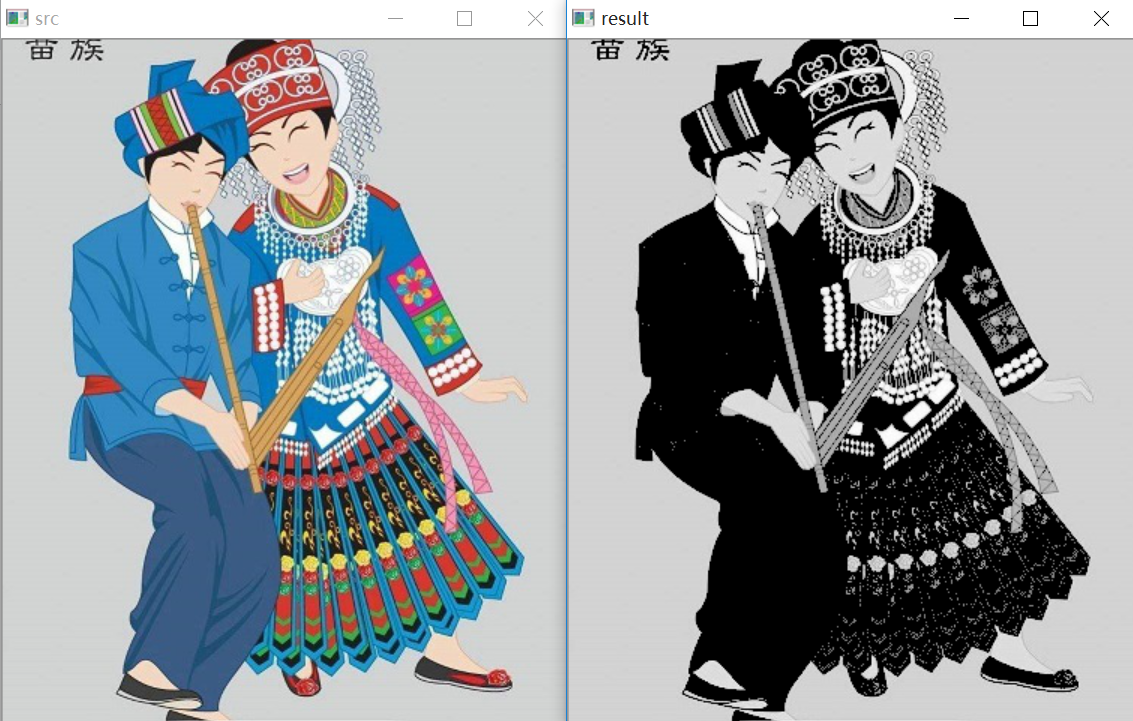#encoding:utf-8
import cv2
import numpy as np
import matplotlib.pyplot as plt

#读取图像
lenna_img = cv2.cvtColor(img,cv2.COLOR_BGR2RGB)
GrayImage=cv2.cvtColor(img,cv2.COLOR_BGR2GRAY)

#阈值化处理
ret,thresh1=cv2.threshold(GrayImage,127,255,cv2.THRESH_BINARY)
ret,thresh2=cv2.threshold(GrayImage,127,255,cv2.THRESH_BINARY_INV)
ret,thresh3=cv2.threshold(GrayImage,127,255,cv2.THRESH_TRUNC)
ret,thresh4=cv2.threshold(GrayImage,127,255,cv2.THRESH_TOZERO)
ret,thresh5=cv2.threshold(GrayImage,127,255,cv2.THRESH_TOZERO_INV)

#显示结果
titles = ['Gray Image','BINARY','BINARY_INV','TRUNC','TOZERO','TOZERO_INV']
images = [GrayImage, thresh1, thresh2, thresh3, thresh4, thresh5]
for i in xrange(6):
plt.subplot(2,3,i+1),plt.imshow(images[i],'gray')
plt.title(titles[i])
plt.xticks([]),plt.yticks([])
plt.show()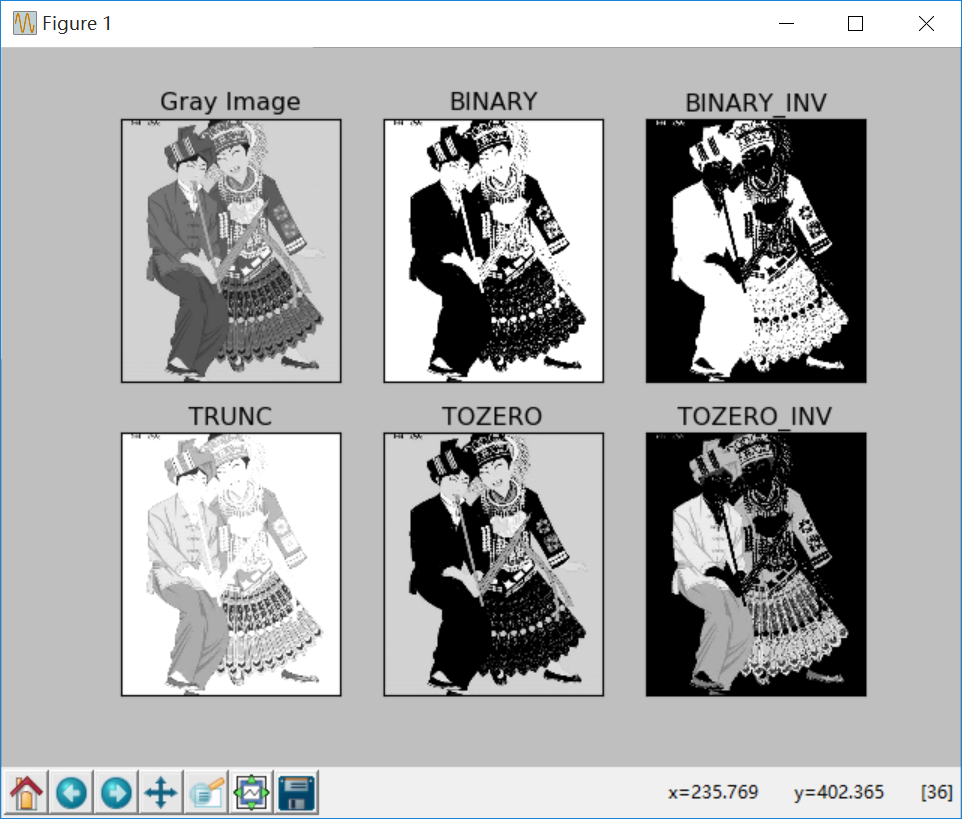（By：Eastmount 2018-10-30 晚上10点 https://blog.csdn.net/Eastmount/）

2019-08-18 10:59:39 weixin_40586929 阅读数 128
• ###### 学习OpenCV3.2+QT5+ffmpeg实战开发视频编辑器视频教程

OpenCV3.2+QT5+ffmpeg实战开发视频编辑器视频培训课程概况：教程中会讲解到基于opencv视频和摄像机录制、播放和播放进度控制，多视频图像合并、多视频图像融合、剪切、视频亮度、对比度、尺寸（近邻插值（手动实现），双线性插值，图像金字塔）、颜色格式（灰度图，二值化（阈值）），旋转镜像，视频裁剪（ROI），视频水印（ROI+weight），导出处理后的视频（包含音频，使用ffmpeg工具对音频进行抽取、剪切和终于opencv处理的视频合并）。

19946 人正在学习 去看看 夏曹俊

Opencv中就有cv2.threshold() 可以做阈值处理。

• cv2.THRESH_BINARY（黑白二值）
• cv2.THRESH_BINARY_INV（黑白二值反转）
• cv2.THRESH_TRUNC （得到的图像为多像素值）
• cv2.THRESH_TOZERO (渐变至1)
• cv2.THRESH_TOZERO_INV (渐变至0)

import cv2

ret,thresh1 = cv2.threshold(img,1,255,cv2.THRESH_BINARY)
ret,thresh2 = cv2.threshold(img,127,255,cv2.THRESH_BINARY_INV)
ret,thresh3 = cv2.threshold(img,127,255,cv2.THRESH_TRUNC)
ret,thresh4 = cv2.threshold(img,127,255,cv2.THRESH_TOZERO)
ret,thresh5 = cv2.threshold(img,127,255,cv2.THRESH_TOZERO_INV)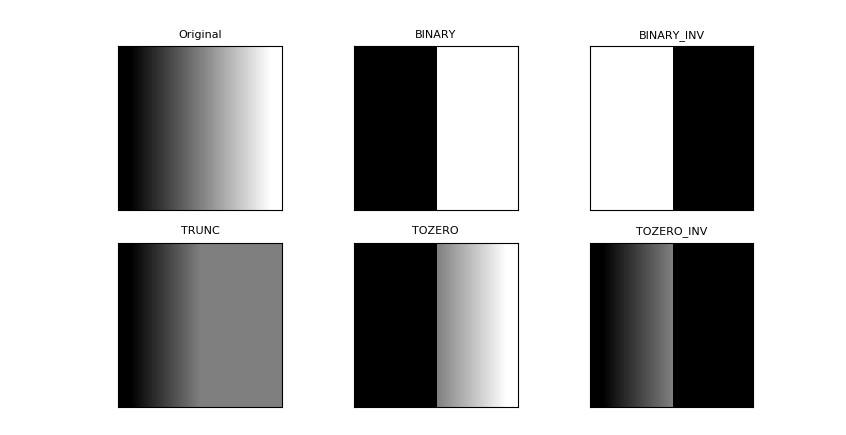2015-07-09 09:03:17 on2way 阅读数 59991
• ###### 学习OpenCV3.2+QT5+ffmpeg实战开发视频编辑器视频教程

OpenCV3.2+QT5+ffmpeg实战开发视频编辑器视频培训课程概况：教程中会讲解到基于opencv视频和摄像机录制、播放和播放进度控制，多视频图像合并、多视频图像融合、剪切、视频亮度、对比度、尺寸（近邻插值（手动实现），双线性插值，图像金字塔）、颜色格式（灰度图，二值化（阈值）），旋转镜像，视频裁剪（ROI），视频水印（ROI+weight），导出处理后的视频（包含音频，使用ffmpeg工具对音频进行抽取、剪切和终于opencv处理的视频合并）。

19946 人正在学习 去看看 夏曹俊
• 图像的阈值处理一般使得图像的像素值更单一、图像更简单。阈值可以分为全局性质的阈值，也可以分为局部性质的阈值，可以是单阈值的也可以是多阈值的。当然阈值越多是越复杂的。下面将介绍opencv下的三种阈值方法。

## （一）简单阈值

• cv2.THRESH_BINARY（黑白二值）
• cv2.THRESH_BINARY_INV（黑白二值反转）
• cv2.THRESH_TRUNC （得到的图像为多像素值）
• cv2.THRESH_TOZERO
• cv2.THRESH_TOZERO_INV

import cv2
import matplotlib.pyplot as plt

ret,thresh1 = cv2.threshold(img,127,255,cv2.THRESH_BINARY)
ret,thresh2 = cv2.threshold(img,127,255,cv2.THRESH_BINARY_INV)
ret,thresh3 = cv2.threshold(img,127,255,cv2.THRESH_TRUNC)
ret,thresh4 = cv2.threshold(img,127,255,cv2.THRESH_TOZERO)
ret,thresh5 = cv2.threshold(img,127,255,cv2.THRESH_TOZERO_INV)
titles = ['img','BINARY','BINARY_INV','TRUNC','TOZERO','TOZERO_INV']
images = [img,thresh1,thresh2,thresh3,thresh4,thresh5]
for i in range(6):
plt.subplot(2,3,i+1),plt.imshow(images[i],'gray')
plt.title(titles[i])
plt.xticks([]),plt.yticks([])
plt.show()## （二）自适应阈值：

• 第一个原始图像
• 第二个像素值上限
• 第四个值的赋值方法：只有cv2.THRESH_BINARY 和cv2.THRESH_BINARY_INV
• 第五个Block size:规定领域大小（一个正方形的领域）
• 第六个常数C，阈值等于均值或者加权值减去这个常数（为0相当于阈值 就是求得领域内均值或者加权值）
这种方法理论上得到的效果更好，相当于在动态自适应的调整属于自己像素点的阈值，而不是整幅图像都用一个阈值。

mport cv2
import matplotlib.pyplot as plt

ret,th1 = cv2.threshold(img,127,255,cv2.THRESH_BINARY)
cv2.THRESH_BINARY,11,2) #换行符号 \
cv2.THRESH_BINARY,11,2) #换行符号 \
images = [img,th1,th2,th3]
plt.figure()
for i in xrange(4):
plt.subplot(2,2,i+1),plt.imshow(images[i],'gray')
plt.show()## （三）Otsu’s二值化import cv2
import matplotlib.pyplot as plt

#简单滤波
ret1,th1 = cv2.threshold(img,127,255,cv2.THRESH_BINARY)
#Otsu 滤波
ret2,th2 = cv2.threshold(img,0,255,cv2.THRESH_BINARY+cv2.THRESH_OTSU)
print ret2
plt.figure()
plt.subplot(221),plt.imshow(img,'gray')
plt.subplot(222),plt.hist(img.ravel(),256)#.ravel方法将矩阵转化为一维
plt.subplot(223),plt.imshow(th1,'gray')
plt.subplot(224),plt.imshow(th2,'gray')print ret2 得到的结果为122。可以看出似乎两个结果并没有很明显差别（素材也不太好弄~_~!），主要是两个阈值（127与122）太相近了，如果这两个隔得很远那么会很明显的。

2019-11-08 12:18:54 qingfengxiaosong 阅读数 30
• ###### 学习OpenCV3.2+QT5+ffmpeg实战开发视频编辑器视频教程

OpenCV3.2+QT5+ffmpeg实战开发视频编辑器视频培训课程概况：教程中会讲解到基于opencv视频和摄像机录制、播放和播放进度控制，多视频图像合并、多视频图像融合、剪切、视频亮度、对比度、尺寸（近邻插值（手动实现），双线性插值，图像金字塔）、颜色格式（灰度图，二值化（阈值）），旋转镜像，视频裁剪（ROI），视频水印（ROI+weight），导出处理后的视频（包含音频，使用ffmpeg工具对音频进行抽取、剪切和终于opencv处理的视频合并）。

19946 人正在学习 去看看 夏曹俊

A.图像处理：对输入的图像做某种变换，输出仍然是图像，基本不涉及或者很少涉及图像内容的分析。比较典型的有图像变换，图像增强，图像去噪，图像压缩，图像恢复，二值图像处理等等。基于阈值的图像分割也属于图像处理的范畴。一般处理的是单幅图像。

B.图像分析：对图像的内容进行分析，提取有意义的特征，以便于后续的处理。处理的仍然是单幅图像。

C.计算机视觉：对图像分析得到的特征进行分析，提取场景的语义表示，让计算机具有人眼和人脑的能力。这时处理的是多幅图像或者序列图像，当然也包括部分单幅图像。

关于图像处理，图像分析和计算机视觉的划分并没有一个很统一的标准。一般的来说，图像处理的书籍总会或多或少的介绍一些图像分析和计算机视觉的知识，比如冈萨雷斯的数字图像处理。而计算机视觉的书籍基本上都会包括图像处理和图像分析，只是不会介绍的太详细。其实图像处理，图像分析和计算机视觉都可以纳入到计算机视觉的范畴：图像处理->低层视觉（low level vision），图像分析->中间层视觉（middle level vision），计算机视觉->高层视觉（high level vision。这是一般的计算机视觉或者机器视觉的划分方法。

2017-08-01 00:16:42 pianzang5201 阅读数 309
• ###### 学习OpenCV3.2+QT5+ffmpeg实战开发视频编辑器视频教程

OpenCV3.2+QT5+ffmpeg实战开发视频编辑器视频培训课程概况：教程中会讲解到基于opencv视频和摄像机录制、播放和播放进度控制，多视频图像合并、多视频图像融合、剪切、视频亮度、对比度、尺寸（近邻插值（手动实现），双线性插值，图像金字塔）、颜色格式（灰度图，二值化（阈值）），旋转镜像，视频裁剪（ROI），视频水印（ROI+weight），导出处理后的视频（包含音频，使用ffmpeg工具对音频进行抽取、剪切和终于opencv处理的视频合并）。

19946 人正在学习 去看看 夏曹俊

• cv2.THRESH_BINARY

• cv2.THRESH_BINARY_INV

• cv2.THRESH_TRUNC

• cv2.THRESH_TOZERO

• cv2.THRESH_TOZERO_INV

#coding:utf-8
import cv2
import numpy as np
from matplotlib import pyplot as plt

ret, thresh1 = cv2.threshold(img,127,255,cv2.THRESH_BINARY)
ret, thresh2 = cv2.threshold(img,127,255,cv2.THRESH_BINARY_INV)
ret, thresh3 = cv2.threshold(img,127,255,cv2.THRESH_TRUNC)
ret, thresh4 = cv2.threshold(img,127,255,cv2.THRESH_TOZERO)
ret, thresh5 = cv2.threshold(img,127,255,cv2.THRESH_TOZERO_INV)

titles = ['Original Image','BINARY','BINARY_INV','TRUNC','TOZERO','TOZERO_INV']
images = [img, thresh1, thresh2, thresh3, thresh4, thresh5]

for i in xrange(6):
plt.subplot(2,3,i+1),plt.imshow(images[i],'gray')
plt.title(titles[i])
plt.xticks([]),plt.yticks([])

plt.show()